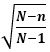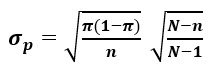# Finite Population Correction factor

The Finite Population Correction Factor, sometimes just called the FPC factor, is used when the sample size is large relative to the population size. For most situations, the population is so large, typical sample sizes are far too small to worry about the need for the FPC.

The guidance is that we need to use the FPC when the ratio of the sample size n to the population size N is greater than 5%. For example, if the population size is 300 and the sample size is 30, we have a ratio of 10% and thus need to use the FPCF.

The most common formula for calculating the FPC isAs the population N gets large compared to the sample n, the FPC tends toward a value of 1.

If it is needed, we use the FPCF to adjust the Standard Error of the analysis at hand. We do that by a simple multiplication of the Standard Error by the FPC value.

If we are working with a sample mean, we adjust the Standard Error of the Mean by multiplying it by the FPC. It can be used to adjust the standard error when we are finding critical values such as for tests of hypothesis and confidence intervals.If we are working with a sample proportion, we multiply the Standard Error of the Proportion by the FPC.This is my Excel solution to a typical problem. Note I calculate the FPC factor first in cell B9 to simplify the process and then multiply it by the standard error in B10.

On the bottom, I show the same problem worked without using the FPC factor and you can see the change on the answers is slight because the FPC is close to 1.